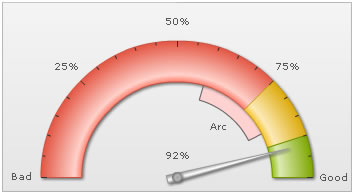The angular gauge allows you to add the following trend elements on the gauge:

Here we'll see how to add these and configure the same.

To add a simple trend point (say last year's customer satisfaction index as 79%), you'll need to add the following to XML:

`<trendpoints>  <point startValue="79" displayValue="Previous" color="666666" thickness="2" alpha="100"/></trendpoints>`
```"trendpoints": {
"point": [
{
"startvalue": "79",
"displayvalue": "Previous",
"color": "666666",
"thickness": "2",
"alpha": "100"
}
]
}```

Here, we've first added the <trendpoints> element, which can contain any number of trend-points for the chart. Now, for each trend point, you need to add a <point> element with startValue/endValue as the value and other cosmetic properties defined.

The above trend point will show up on the chart as under: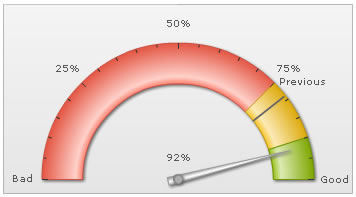You can have the following properties for <point> element:

 Attribute Name Type / Range Description startValue Number The starting value for the trend-point. Say, if you want to plot a trend arc from value 102 to 109, the startValue will be 102. endValue Number The ending value for the trend-point. Say, if you want to plot a trend-arc from value 102 to 109, the endValue will be 109. displayValue String Display label for the trend-point/trend-arc. If you do not specify a value, the chart displays the numeric value. valueInside Boolean (0/1) Whether to display the trend-point value inside the gauge? color Color Code Color of the trend point and its associated text. thickness Number (Pixels) If you've opted to show the trend as a point, this attribute lets you define the thickness of trend line. showBorder Boolean (0/1) If you're drawing a trend-arc, you can set its border on using this attribute. borderColor Color Code Lets you define the border color for trend-arc. radius Number (Pixels) Radius for trend point/outer side of trend arc. innerRadius Number (Pixels) In case of trend-arc, radius for inner side of arc. dashed Boolean (0/1) If you're showing the trend point as line, this attribute lets you control whether the line will be plotted as dashed? dashLen Number (pixels) Length of each dash, if trend-point is plotted as dashed line. dashGap Number (pixels) Length of each dash gap, if trend-point is plotted as dashed line. useMarker Boolean (0/1) You can opt to show a triangular marker for each trend-point by setting this attribute to 1. markerColor Color Code Fill color of marker. markerBorderColor Color Code Border color of marker. markerRadius Number (pixels) Radius of the marker. markerTooltext String You can define tooltip for marker using this marker.

If we wanted to customize the radius for this trend point, we can write:

`<point startValue='79' ... radius='150' innerRadius='95'/>`
```"point": [
{
"startvalue": "79",
...
}
]```

Here we're using our own pixel based outer and inner radius for the trend point. This will result in: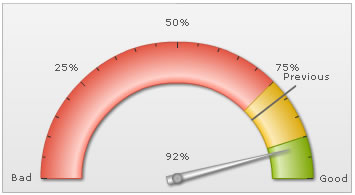You can also place the value inside by setting:

`<point ... valueInside='1' ...>`
```"point": [
{
...
"valueinside": "1",
...
}
]```

This will result in: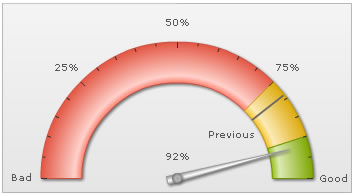The line can also be drawn as dashed one by setting:

`<point ... dashed='1' dashLen='3' dashGap='3' ..>`
```"point": [
{
"dashed": "1",
"dashlen": "3",
"dashgap": "3"
}
]```

This will result in: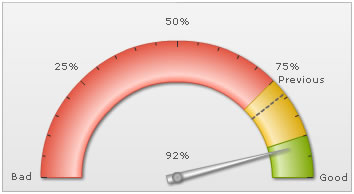If you wish to increase the space between trend line and its value, you can set it in:

<chart ... trendValueDistance='25' ..> (or a value in pixels)

This might often be necessary when you've long trend labels.

Showing trend-markers

For each trend point, you can also show a triangular marker using:

`<point startValue='79' displayValue='Previous' color='666666' useMarker='1' markerColor='F1f1f1' markerBorderColor='666666' markerRadius='7'/>`
```"point": [
{
"startvalue": "79",
"displayvalue": "Previous",
"color": "666666"
"usemarker": "1",
"markercolor": "F1F1F1",
"markerbordercolor": "666666",
}
]```

This will result in: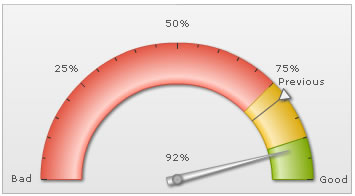You can also add a tooltip to this marker using:

`<point ... markerTooltext='Previous year index: 79%' ...>`
```"point": [
{
...
"markertooltext": "Previous year index: 79%"
...
}
]```

This will result in: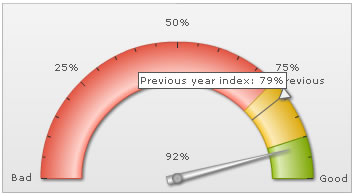Creating trend-arcs

You can also create trend-arcs to represent a range of values, instead of a single value. Shown below is a simple XML:

`<point startValue='59' endValue='85' displayValue='Arc' valueInside='1' color='FF0000'  alpha='30' />`
```"point": [
{
"startvalue": "59",
"endvalue": "85",
"displayvalue": "Arc"
"valueinside": "1",
"color": "FF0000",
"markeralpha": "30"
}
]```

Here, we've added both startValue and endValue to create a trend arc. Also, we've specified the cosmetics. This will result in: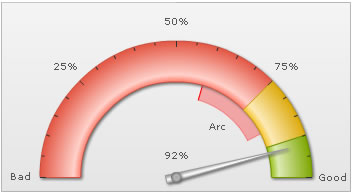You can customize its radius as under:

`<point startValue='59' endValue='85' color='FF0000' alpha='20' radius='130' innerRadius='5' showBorder='0'/>`
```"point": [
{
"startvalue": "59",
"endvalue": "85",
"color": "FF0000",
"alpha": "20",
"showborder": "0"
}
]```

This will result in: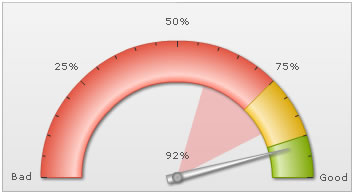You can opt to show border for the arc using:

`<point ... showBorder='1' borderColor='666666' ...>`
```"point": [
{
"showborder": "1"
"bordercolor": "666666"
}
]```

This will result in: